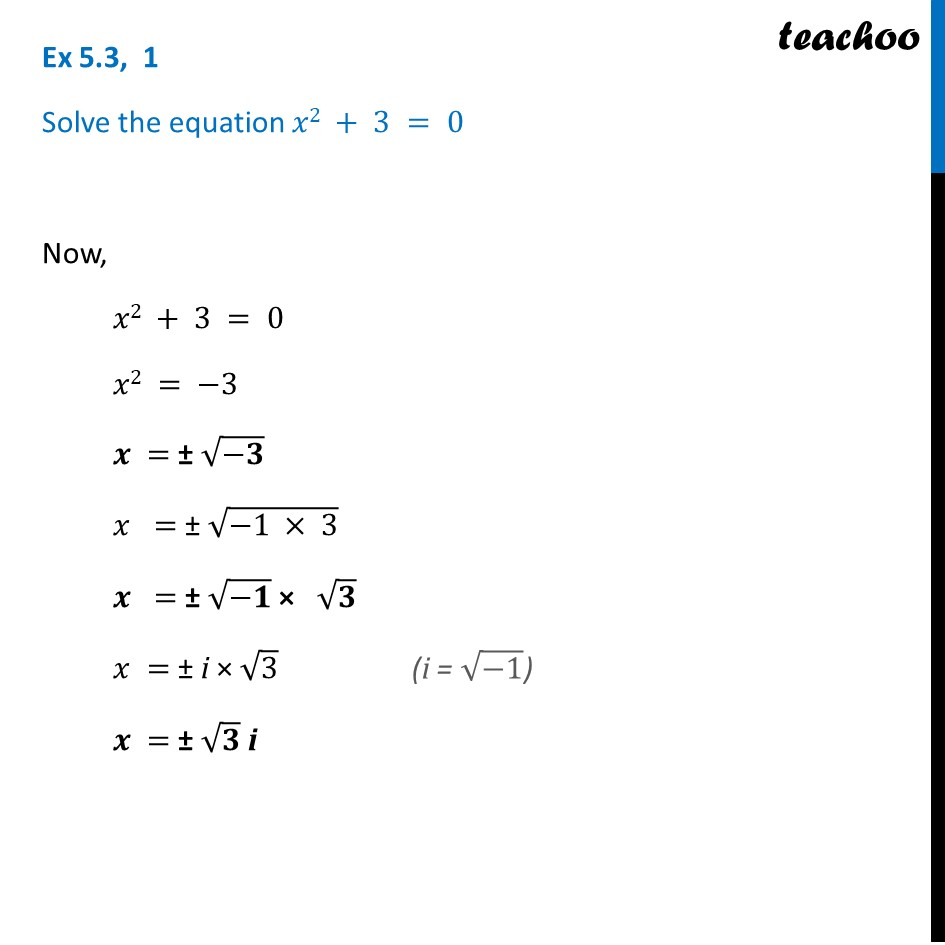Chapter 4 Class 11 Complex Numbers
Serial order wiseLearn in your speed, with individual attention - Teachoo Maths 1-on-1 Class

### Transcript

Question 1 Solve the equation 𝑥2 + 3 = 0 Now, 𝑥2 + 3 = 0 𝑥2 = −3 𝒙 = ± √(−𝟑) 𝑥 = ± √(−1 × 3) 𝒙 = ± √(−𝟏) × √𝟑 𝑥 = ± i × √3 𝒙 = ± √𝟑 i